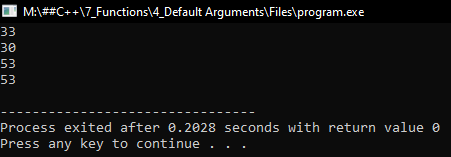# Default Arguments in C++

## Default Arguments in C++ with Examples:

In this article, I am going to discuss Default Arguments in C++ Language with examples. Please read our previous article, where we discussed Function Template in C++ with examples.

##### Default Arguments in C++:

Let us understand default arguments in C++ with an example. We have taken two functions for the explanation,

return x + y;
}
int add(int x, int y, int z){
return x + y + z;
}

Here both the function names are ‘add’ but the first function is taking 2 integers and the second function is taking 3 integers. So, they are overloaded functions with the same name and a different number of arguments.

Now if we write ‘add(2, 5)’ then it will call the first function and if we write ‘add(3,4,5)’ then it will call the second function that is taking 3 arguments. Can we combine these two functions and write a single function? Let us see,

int add(int x, int y, int z = 0){
return x + y + z;
}

Here we are taking the ‘z’ parameter as ‘0’, what does it mean? This is the default argument. ‘z’ is a default argument. Here we have given some default values to the ‘z’ parameter. Now if we write ‘add(2, 5)’ then this will call the above function with the parameter values as ‘x = 2’, ‘y = 5’ and ‘z = 0’ as we have not mentioned ‘z’ in the call so it will take the default value.

And if we write ‘add(2,5,7)’ then the values will be ‘x = 2’, ‘y = 5’ and ‘z = 0’. So, this means the ‘z’ parameter is optional. It is up to the user. If the ‘z’ value is given by the user then it will take that otherwise it will take the default value that is ‘0’ in this case.

So, we have combined the above two overloaded functions into one function. This is called the Default Argument in C++. So, assigning some default values to the argument is the default argument function and the benefit of a default argument is we can combine the overloaded function and write one single function. This is a very powerful feature of C++ and it is friendly for the programmer. Now one important thing about this one, suppose we have a function ‘fun’,

int fun(int a, int b, int c, int d){
}

In this function, we can make ‘d’ as default parameter or ‘c’ and ‘d’ as default parameter or ‘b’, ‘c’ and ‘d’ as default parameter but we cannot make ‘a’ as default parameter and skip to ‘b’ making this as mandatory. This is not possible. We should start making the Default arguments from right to left without skipping any parameter. Now let us look at the complete program.

##### Program to understand Default Arguments in C++
```#include <iostream>
using namespace std;
int Sum(int x, int y, int z = 0)
{
return x + y + z;
}

int Max(int a = 0, int b = 0, int c = 0)
{
return a > b && a > c ? a : (b > c ? b : c);
}

int main()
{
cout << Sum(10, 20, 3) << endl;
cout << Sum(10, 20) << endl;
cout << Max(13, 22, 53) << endl;
cout << Max(22, 53) << endl;
return 0;
}
```
###### Output:#### Default Arguments Frequently Asked Questions

No.

##### Default values should be filled from which side?

Default values for formal arguments must be foibles from the right side without skipping any parameter.